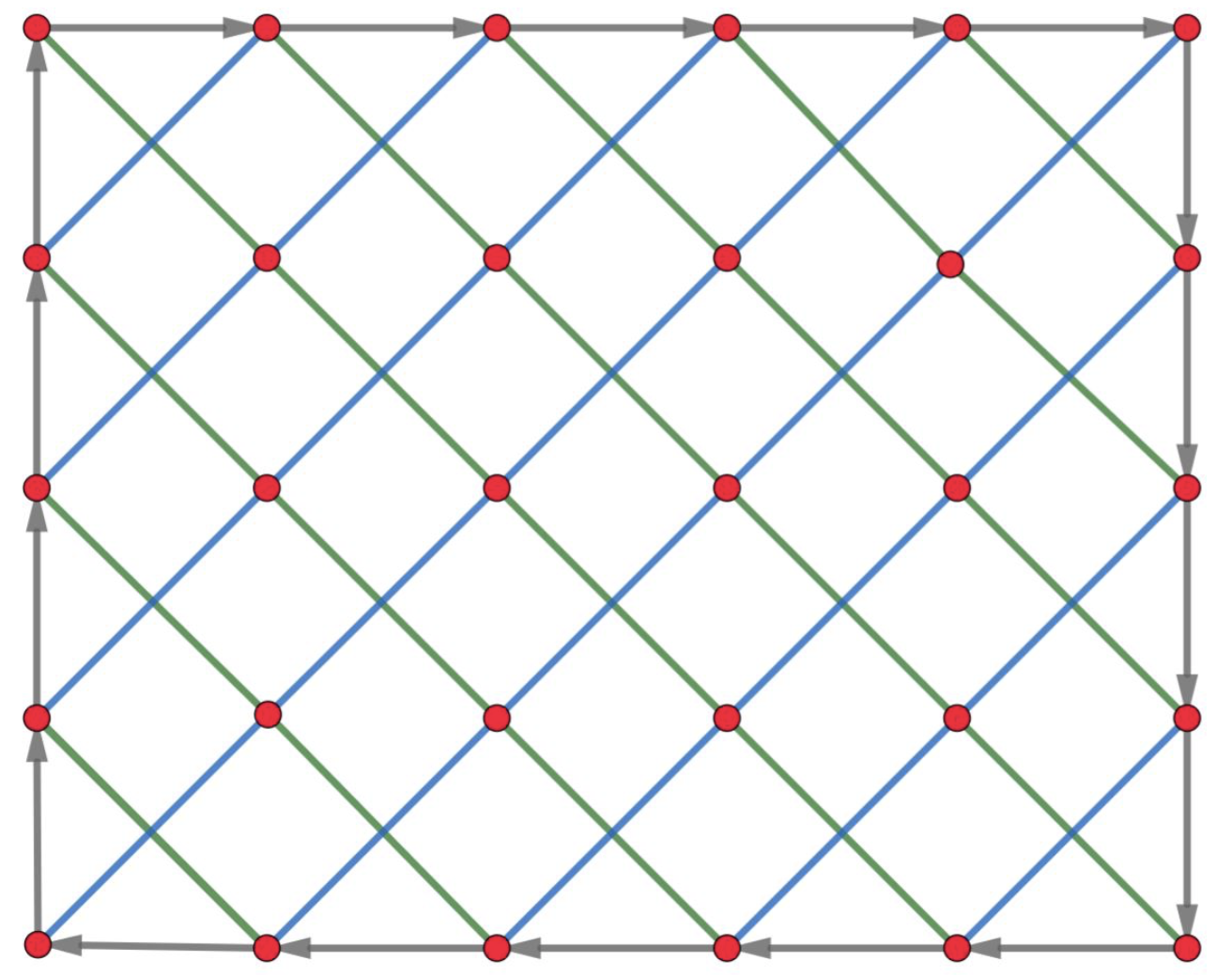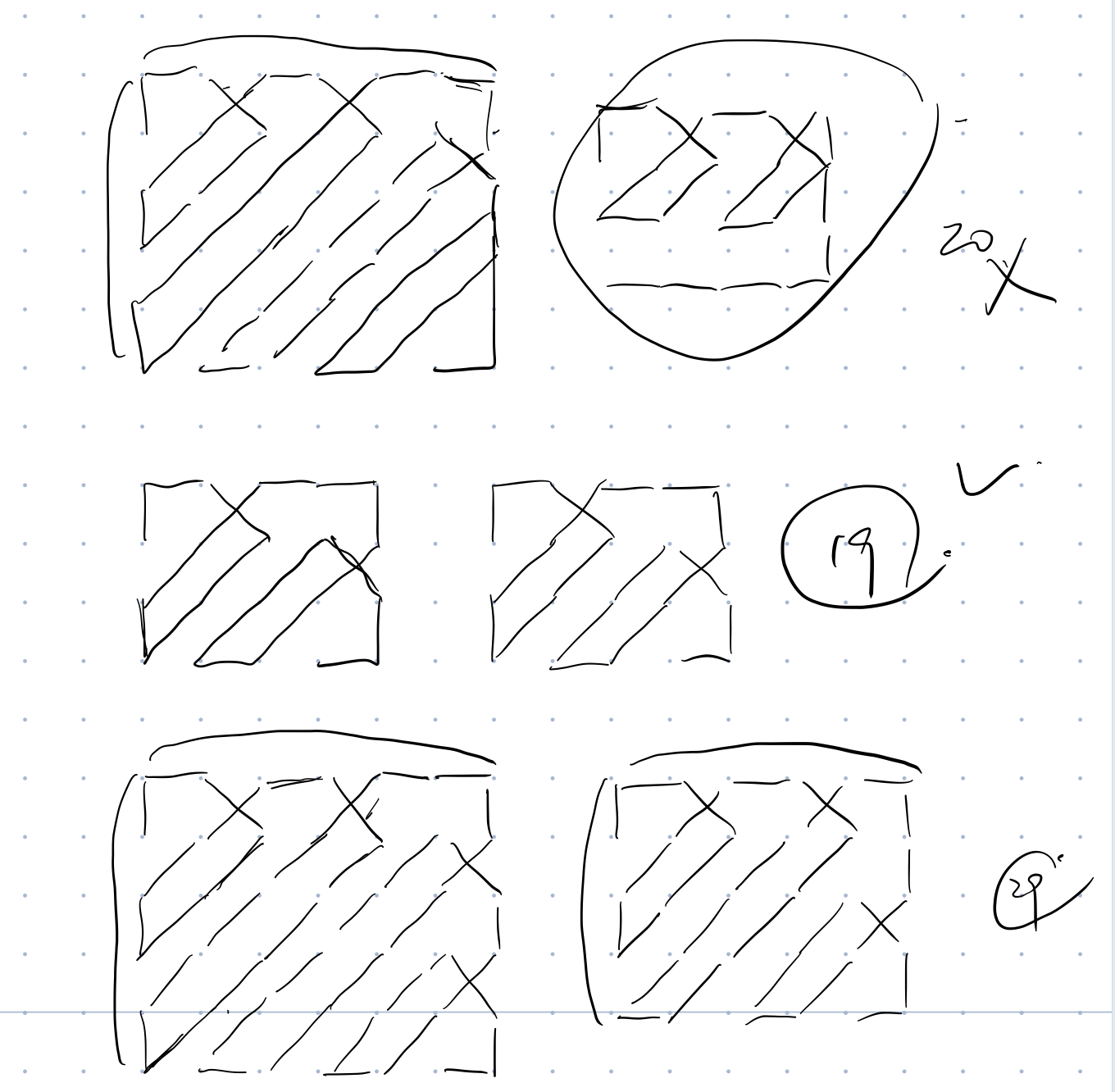# 「ACM-XCPC」2020-学军信友邀请赛(Updating)

## IOI赛制确实快乐但这和我骗不到分有什么关系呢.jpg

Posted by Culaccino on April 5, 2020

## A 核酸检测## B 齐心抗疫

#include<cstdio>
#include<vector>
#include<cmath>
#define pb push_back

int n;
int a;
vector<int>G;
int dx,dy;

int dfs(int now,int pre,int* d){
int res=now;
for(int i=0;i<G[now].size();i++){
int nxt=G[now][i];
if(nxt==pre)continue;
d[nxt]=d[now]+1;
int tmp=dfs(nxt,now,d);
if(d[tmp]>d[res]) res=tmp;
}
return res;
}

int main(){
scanf("%d",&n);
for(int i=1;i<=n;i++) scanf("%d",&a[i]);
int tx,ty;
for(int i=1;i<=n-1;i++){
scanf("%d%d",&tx,&ty);
G[tx].pb(ty);G[ty].pb(tx);
}
int x=dfs(1,0,dy);
int y=dfs(x,0,dx);
fill(dy,dy+50010,0);dfs(y,0,dy);
int ans=-1;
for(int i=1;i<=n;i++){
ans=max(ans,a[i]*max(dx[i],dy[i]));
}
printf("%d\n",ans);
}


## D 抗议斗争

(比赛的时候果然题目读错了（x

①能够通过1e5复杂度的部分：首先是对于h[m]的部分，易得若m为奇数，则h[m]必为1，因为此时在后序回合中双方均只能每次消耗1，且人类方能够先用完；若m为偶数，则h[m]必为偶数，若为奇数则当到病毒回合时会剩余奇数，则对于病毒而言会到达必胜点（病毒只需此时消耗1即可胜利），且由于每一步最优，有 $h_{2m}=2h_m\ \ \ \ \$ 即可得到$h_m=lowbit(m)$

———以下是待update的部分qwq———

②能通过1e11的部分：分块处理，让复杂度达到O(√n)